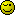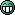# Simultaneous Equation Help Please :)

#### Guitarman0

Associate
Hi there,

I have a simultaneous equation question that has been driving me crazy and I just can't see what I am doing wrong.

x^2 - 2y^2 = 8

x + 2y = 8

So, here is what I did:

I squared the 2nd equation to get

x^2 + 2y^2 = 64

I then added the new equation and the x^2 - 2y^2 = 8 equation to get

2x^2 = 72

I then divided both sides by 2 to get

x^2 = 36

and then square rooted x to get

x = 6

However, this doesn't work for both equations and the answers in the back of the book say x = 4, y = 2 OR x = -20, y = 14.

Could someone please show me where I am going wrongCheers

#### Haircut

Soldato
Squaring the second equation doesn't give x^2 + 2y^2 = 64

It gives x^2 + 4xy + 4y^2 = 64

#### |Thomas|

Associate
^ this, a better approach would be to rearrange the second equation so that you have just one of the variables on one side, i.e.:

x = 8 - 2y
or y = 0.5*(8-x)

THEN square both sides and substitute that into the first equation. I'm not going to do that for you though, that's your job#### Westyfield2

Soldato
Squaring the second equation doesn't give x^2 + 2y^2 = 64

It gives x^2 + 4xy + 4y^2 = 64

Bingo!

#### Shayper

Permabanned
Simple:

x + 2y = 8

Rearrange the second equation to make x = 8 - 2y

Substitute this into the first equation to make (8 - 2y)^2 - 2y^2 = 8

Expand the brackets to give (8 - 2y)(8 - 2y) - 2y^2 = 8

Multiply out to give 64 - 32y + 4y^2 - 2y^2 = 8

Collect like terms to give 2y^2 - 32y + 56 = 0

Factorise to give 2(y^2 - 16y + 28) = 0

Divide both sides by 2 to give (y^2 - 16y + 28) = 0

Factorise again to give (y - 14)(y - 2) = 0

So therefore y = 14 or y = 2

Substitute both values of y into the original second equation to give

x + 28 = 8 or x + 4 = 8

Therefore when y = 14 x = -20

And when y = 2 x = 4

Sorted#### Guitarman0

Associate
Thanks for the replies guys, I got it sorted now. I tried the same method as you gave shayper earlier but made the mistake of thinking 2y x 2y = 2y^2 (noob mistake lol).

Thanks a lot#### Shayper

Permabanned
HahaNo worries mateThat's what a maths degree is for... I can't wait to go back!#### goldilocks

Associate
simultaneous equations are a waste of time because
there is only one profession where you'd ever need to be able to find 'x'.
pirate.

#### mattyg

Soldato
20 yrs ago that would have been easy.

Now none of it makes any sense.